## Example Questions

← Previous 1 3

### Example Question #1 : How To Find The Decimal Equivalent Of A Fraction

0.3 < 1/3

4 > √17

1/1/8

–|–6| = 6

Which of the above statements is true?

4 > √17

1/< 1/8

–|–6| = 6

0.3 < 1/3

0.3 < 1/3

Explanation:

The best approach to this equation is to evaluate each of the equations and inequalities.  The absolute value of –6 is 6, but the opposite of that value indicated by the “–“ is –6, which does not equal 6.

1/2 is 0.5, while 1/8 is 0.125 so 0.5 > 0.125.

√17 has to be slightly more than the √16, which equals 4, so“>” should be “<”.

Finally, the fraction 1/3 has repeating 3s which makes it larger than 3/10 so it is true.

### Example Question #1 : Decimals With Fractions

Quantitative Comparison: Compare Quantity A and Quantity B, using additional information centered above the two quantities if such information is given.

10 < n < 15

Quantity A          Quantity B

7/13                    4/n

Quantity A is greater.

Quantity B is greater.

The answer cannot be determined from the information given.

The two quantities are equal.

Quantity A is greater.

Explanation:

To determine which quantity is greater, we must first determine the range of potential values for Quantity B. Let's call this quantity m. This is most efficiently done by dividing 4 by the highest and lowest possible values for n.

4/10 = 0.4

4/15 = 0.267

So the possible values for m are 0.267 < m < 0.4

Now let's find the value for 7/13, to make comparison easier.

7/13 = 0.538

Given this, no matter what the value of n is, 7/13 will still be a higher proportion, so Quantity B is greater.

### Example Question #2 : Decimals With Fractions

Quantity A: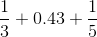Quantity B: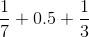The two quantities are equal.

Quantity A is greater.

Quantity B is greater.

The relationship cannot be determined from the given information.

Quantity B is greater.

Explanation:

The GRE test now has a built-in calculator.  Simply convert the fractions to decimals and compare:

Quantity A = 0.333 +0.43 + 0.2 = 0.963

Quantity B = 0.1429 + 0.5 + 0.3333 = 0.976

Thus, Quantity B is larger.

### Example Question #2 : How To Find The Decimal Equivalent Of A Fraction

How much less is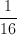than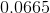?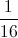is greater than.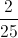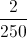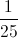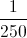Explanation: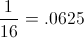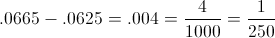### Example Question #3 : How To Find The Decimal Equivalent Of A Fraction

The ogre under the bridge eatsof a pizza and then throws the rest of the pizza to the rats. The rats eatof what is left. What fraction of the pizza is left when the rats are done?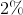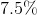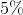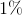Explanation:

1/5 of the pizza is left after the ogre eats his share. The rats eat 3/4 of that, so 1/4 of 1/5 of the pizza is left.

1/4 * 1/5 = 1/20 = 5%

### Example Question #161 : Fractions

Which of the following numbers is between 1/5 and 1/6?

0.13

0.19

0.22

0.25

0.16

0.19

Explanation:

Long division shows that 1/5 = 0.20 and 1/6 = 0.16666...  0.13 < 0.16 < 1/6 < 0.19 < 1/5 < 0.22 < 0.25.

### Example Question #162 : Fractions

Trevor, James, and Will were each given a candy bar. Trevor ate 7/12 of his and Will ate 20% of his. If James ate more than Will and less than Trevor, what amount could James have eaten?

8/9

3/5

2/7

1/10

9/15

2/7

Explanation:

Turn Trevor and Will’s amounts into decimals to compare: 20% = 0.20  and 7/12 = 0.5083 rounded. When the answer choices are converted into decimals, 2/7 = 0.2871 is the only value between 0.20 and 0.5083.

### Example Question #3 : Decimals With Fractions

Choose the answer which best expresses the following fraction as a decimal (if necessary, round to the nearest hundredth):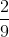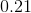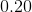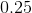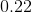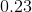Explanation:

To solve this problem, simply divide the numerator by the denomenator. We see that when we try to divide these two numbers that 9 does not go into 2 therefore we need to add a decimal place and a zero to 2. Now we have 2.0 divided by 9 from here we can see that .2 times 9 gives us 1.8 which is close to 2. Now we subtract 1.8 from 2 and are left with .2. We repeat this process.

You are left with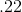repeating, which can be rounded to, so that is the best answer.

### Example Question #4 : Decimals With Fractions

Choose the answer below which best expresses the following fraction as a decimal (round to the nearest hundredth, if necessary):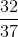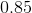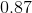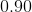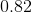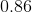Explanation:

To express a fraction as a decimal, simply divide the numerator by the denomenator.  In this case, you divide thirty two by thirty seven. From here add a decimal place and a zero after 32. Now we are able to divide.

Thus yielding: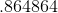repeating.

Round to the nearest hundredth, and you get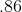because the value in the thousandths place is a four or lower therefore, the value in the hundredths place remains the same.

### Example Question #5 : Decimals With Fractions

Choose the answer below which best expresses the following fraction as a decimal (round to the nearest hundredth, if necessary):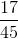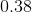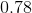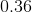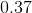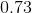Explanation:

To convert, first divide the numerator by the denomenator. For this problem we will need to add a decimal place and a zero to the end of 17 before we divide.

Then, you will yield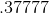repeating.

Then you can round to the nearest hundredth, which will give you your final answer of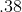.

Since the value in the thousandths place is a seven which is greater than four, we will need to round the value in the hundredths place up to eight.

← Previous 1 3

Tired of practice problems?

Try live online GRE prep today.